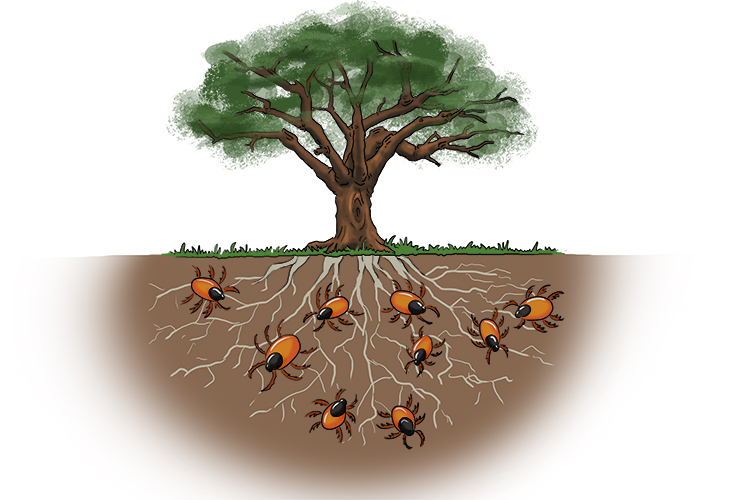# Square root

## (i.e. the root of the number)

The square root has a symbol that is shaped like a tick.

√ This is the symbol of the square root which looks like a tick.

To remind you that the symbol of a square root is a tick, see below:To help you remember what the square root of a number is think of a ROOT OF A TREE covered in ticks (bugs) (see below).

Examples

What is the square root of 9?

We know the tree is 9 but what is its ROOT?

The root of 9 is 3  (3times3=9)

NOTE:

The square root has a symbol, a sort of tick with a line.

root()9=3

We say the square root of 9 equals 3.

Here are the first 10 square roots of numbers up to 100:

 root()1 = 1 root()4 = 2 root()9 = 3 root()16 = 4 root()25 = 5 root()36 = 6 root()49 = 7 root()64 = 8 root()81 = 9 root()100 = 10

NOTE:

These should be easy because if you see the section on multiplication tables it is imperative that a child should know all numbers up to 12 multiplied by themselves.